# Conductance: What is it? (Definition, Units & Formula)

Contents

## What is Conductance?

Conductance (also known as electrical conductance) is defined as the potential for a substance to conduct electricity. Conductance is the measure of how easily electrical current (i.e. flow of charge) can pass through a material. Conductance is the inverse (or reciprocal) of electrical resistance, represented as 1/R.

To have a better understanding of conductance, one must recall the resistance of an object. In a qualitative sense, the resistance tells us how difficult it is for an electrical current to pass. The resistance between two points can be defined in the quantitative sense as the difference in voltage that is needed to carry a unit current across the two specified points.

The resistance of an object is represented as the ratio of the voltage across something to the current passing through it. The resistance is measured in Ohms. The conductance of a component is a determination of how quickly current can flow within the component. Conductance is measured in Siemens (S).

## Conductance Formula and Measuring Units

In electronics, conductance is a measure of the current generated for a given applied voltage by a circuit device. Typically denoted by the letter G, conductance is resistance reciprocal, R. To define the formula, we need to apply Ohm’s law which states that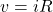from which R can be calculated as

(1)The word conductivity is the opposite of this expression. It is expressed as a current to voltage ratio.

(2)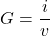Conductance is expressed as G and the measuring unit was “mho”. Later after a few years, researchers replaced the unit with “Siemens” which was denoted by the letter S. When looking at resistance vs conductance – conductance is the inverse of resistance (i.e. 1/resistance), as shown below:

(3)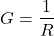## How to calculate Conductance?

Conductance can be calculated with the help of the resistance, current, voltage and conductivity.

For example for a particular circuit element that has a resistance of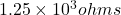, determine the conductance value. We know

(4)On substituting the value of given R and value of conductance can be obtained asThe next example is an electric circuit, in which a 5V input generates a current of 0.3A in a length of wire. According to Ohm’s law V=IR from which resistance can be calculated as

(5)Conductance is reciprocal of resistance. So,

(6)(7)On substituting the values of i and v, one can derive the value of conductance as 0.06 Siemens

Conductance can be calculated from conductivity. Suppose a wire with a round cross-section of radius r and length L is given with known resistivity value of the wire material, conductance G of the wire can be determined. The relationship between G and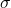is

(8)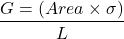where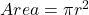For example, if there is an iron rod with a radius of 0.001 meters and length 0.1 meters, calculate the conductance of the rod. Assumeof iron isSiemens/m. The area is calculated usingas. On doing a further substitution, conductance is found to be 324 Siemens.

## Conductivity

Conductivity attributes to the ability of a material to transfer energy and is one of the characteristic property which is used to describe the electromagnetic properties of materials. It quantifies the effect of matter on current flow in response to an electric field. It is also understood as a material property that determines the density of the conductive current in response to an applied electric field. A conductor’s conductance depends on several factors including its form, dimensions and the material’s property called its conductivity.

Conductivity is expressed asand measured as Siemens per meter. The opposite of resistance is conductivity. Since resistance is the opposite of the current flow, conductance is the amount of current a material may carry out. For example, a low-resistance material is highly conductive and vice-versa. Conductivity is also known as specific conductance. There are different types of conductivity namely Electric, Thermal, Ionic and Acoustic.

## Conductivity vs Conductance

The degree to which a given material conducts electricity is known as conductivity. It is calculated as the ratio of the current density in the material to the electric field which produces the flow of current. It calculates the amount of energy that can actually move through a system like in the Electrical circuit. The ability of an object to transmit heat, sound or electricity is known as conductivity.

Conductance tells about to what extent an object conducts electricity, expressed in units of Siemens. It is measured as the ratio of the current that flows to the existing potential difference. It depends on the dimensions of the conductor. Conductance refers to the amount of energy transmitted through a material or substance.

## Applications of Electrical Conductivity Measurement

### Electrical Conductivity and Agriculture

Knowing the electrical conductivity of soil is extremely important for the health and growth of crops when it comes to the agricultural industry. Farmers, as well as growers, are often concerned with monitoring soil phosphates, nitrates, calcium, and potassium because these nutrients are essential for successful plant growth.

Checking the soil’s Electrical Conductivity (EC) will help growers keep track of all of the nutrients in their soil. EC can indicate the number of nutrients in the soil and help growers determine if their soil needs more nutrients or if there are too many nutrients. Using sensors for assessing soil’s apparent electrical conductivity (EC) provides a way to overcome these constraints.

### Electrical Conductivity and Water Treatment

Electrical Conductivity (EC) plays a major role in different applications of water quality. In wastewater treatment, EC is assessed to ensure that the salinity of wastewater outgoing is equal to the body of water it is discharged into. The release of water which has an extremely high or low salinity can adversely affect the health of aquatic life.

### Electrical Conductivity and Electroplating Bath

Conductivity can also impact galvanized waters and is a common check-in industry such as aerospace, automotive, and jewelry. Metal rinse baths are also used to extract residual chemicals from plated items. When this happens, countercurrent rinses help mitigate produced wastewater.

Measuring the conductivity of the plating process at this point decides whether more water is required and needs to be flushed in. Measurements of conductivity can be used to provide useful industry-specific measurements such as total dissolved solids (TDS) and salinity

Want To Learn Faster? 🎓
Get electrical articles delivered to your inbox every week.
No credit card required—it’s 100% free.